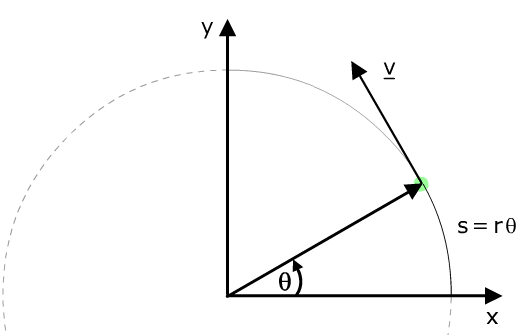# Circular motion.

Acceleration is, by definition, the time rate of change of the velocity. While speed is a scalar, velocity is a vector: velocity has magnitude and direction. (See the module on constant acceleration, and the page on vectors.) In the animation below, the velocity vector is shown as an arrow. It is changing direction, but not changing magnitude.Let's look at the angular position, θ, measured from the positive x axis. The distance travelled from the x axis is the arc with length s = rθ. The speed v, written without a vector bar, is the magnitude of the velocity. By definition, the speed is ds/dt. (See An introduction to calculus.) The radius, r, is constant, so v is r times dθ/dt. v is the rate of change of linear position, and dθ/dt is the rate of change of angular position. We call it the angular velocity and use the symbol ω. So the magnitude of the velocity is rω, and its direction is tangential. If it completes one circle in a period T, then the angle increases by 2π radians, so ω is 2π/T and v  =  rω  =  2πr/T. This is no surprise: it is one circumference per period.

### Airborne automobiles

The animation below is introduced in the Circular Motion module, and is reproduced here for those who might like to view it in slow motion.

The car drives at constant speed over a hill at whose summit the radius of curvature, in the vertical plane, is 30 m, as shown. Let's find the critical speed vcrit at which the car loses contact with the road.

So, at the top of the hill, the weight is the only downwards force, and so the maximum downwards acceleration is g, which, as we saw in the Projectile module, is 9.8 m.s−2. Now the downwards centripital acceleration when the car is at the top of the hill is v2/r, and this acceleration cannot be greater than g. So the critical acceleration and velocity are

acrit  =  vcrit2/r  =  g.
Rearranging this equation gives the critical speed:
vcrit  =  √(rg)  =  17 m.s−1   =  62 k.p.h.
Note that, while in contact with the road, the car is rotating in the vertical plane with angular velocity ω = v/r. At the point near where it loses contact with the road, the forces exerted on the wheels are small, and so too are the torques (which we shall meet when we come to Rotation). Consequently, the car, whilst airborne, continues to rotate at this rate. This rotation adds extra danger to the manoeuvre, which is just one of the reasons for the advice, rarely given in physics, that one should trust the calculation here, rather than perform the experiment.

* Racing cars often have a large foil whose effect, at high speed, is to provide a downwards force and thus allow greater frictional forces for cornering, braking and even acceleration. Some street cars have a miniature version of this foil. These have several possible functions. First, they identify the style of the driver, which may provide a warning to other drivers. Second, they increase drag and petrol consumption, which benefits oil companies (in the short term, at least). Do they have a significant effect in producing a downwards force? Taking the weight of the car as 20 kN, a 10% increase in downwards force would require the foil to exert 2 kN downwards. I do not recommend the following experiment, but if it can exert 2 kN, then it ought to support, elastically, the weight of a few people standing on it.

### What is the acceleration of your room?

This is not an idle puzzle. Aristarchus of Samos and, much later, Copernicus argued the earth orbits the sun. On the other side Aristotle, Ptolemy and many others believed that the sun must go around the earth, because they did not feel the motion of the earth. Who is correct? We do not feel constant velocity (try closing your eyes on a smooth train or plane ride), but we do feel accelerations, both by the forces on us that cause accelerations and by the forces acting in the middle ear when the head accelerates. So, what is your acceleration due to the rotation and orbital motion of the Earth?

You will need to know some values: the period of the Earth's rotation is (slightly less than) 24 hours. The actual value depends on your latitude, but your distance from the Earth's axis is not greater than six thousand kilometres. So

What is the acceleration due to the Earth's rotation about its axis?
To get an idea whether you would feel this, you could express your answer as a fraction of g.

You should do a similar calculation for the acceleration due to the Earth's orbit, which has a radius of 1.5 x 1011 m.

### Components of uniform circular motion

Let's consider a point at vector r undergoing uniform circular motion. Circular, so its magnitude r is constant, and uniform, so its angular displacement θ = ωt. From trignometry, its x and y components are
x  =  r cos θ,    y  =  r sin θ   so:
x  =  r cos ωt,    y  =  r sin ωt
and it's left as an exercise to check that x2 y2 = 0.

### What if the circular motion is not uniform?This work is licensed under a Creative Commons License.